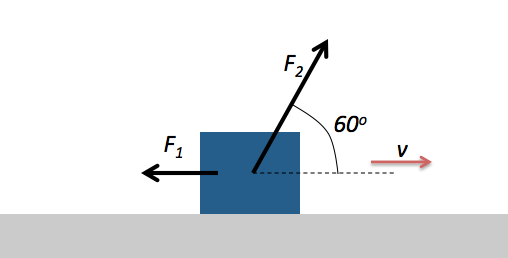Classical Mechanics

# Power input to a systemThe figure above shows constant forces $\vec{F_1}$ and $\vec{F_2}$ acting on a box as the box slides rightward across a frictionless horizontal floor. Force $\vec{F_1}$ acts horizontally leftward with a magnitude of $4 \text{ N} .$ Force $\vec{F_2}$ is applied in a direction that makes a $60^\circ$ angle with the floor with a magnitude of $8 \text{ N}.$ The speed $v$ of the box at a certain instant is $6 \text{ m/s}.$ What is the net power acting on the box?

A skier is pulled by a towrope up a frictionless ski slope that makes a $30^ \circ$ angle with the horizon. The rope moves parallel to the slope at a constant speed of $1 \text{ m/s}.$ The rope does $800 \text{ J}$ of work to the skier as the skier moves a distance of $8 \text{ m}$ up the incline. If instead the rope moved at a constant speed of $2 \text{ m/s}$, while the skier moves the same distance, with how much power would the rope do work to the skier?

A $4 \text{ kg}$ cactus initially at rest accelerates uniformly for $16$ seconds. If the cactus' speed after the acceleration is $16 \text{ m/s} ,$ what is the instantaneous power with which work is done to the cactus, just before the end of its acceleration?

A freight elevator is loaded with a cab with a total mass of $1200 \text{ kg},$ which is required to travel upward $18 \text{ m}$ in $100$ seconds, starting and ending at rest. The elevator's counterweight has a mass of only $1000 \text{ kg},$ so the elevator motor must help. With how much power must the motor do work to the cab?

The gravitational acceleration is $g= 10 \text{ m/s}^2.$

A $80 \text{ kg}$ block is pulled at constant speed of $7.0 \text{ m/s}$ across a horizontal floor by an applied force of magnitude $4 \text{ N}$ in a direction that makes a $60 ^\circ$ angle with the floor. With how much power does the force do work on the block?

×